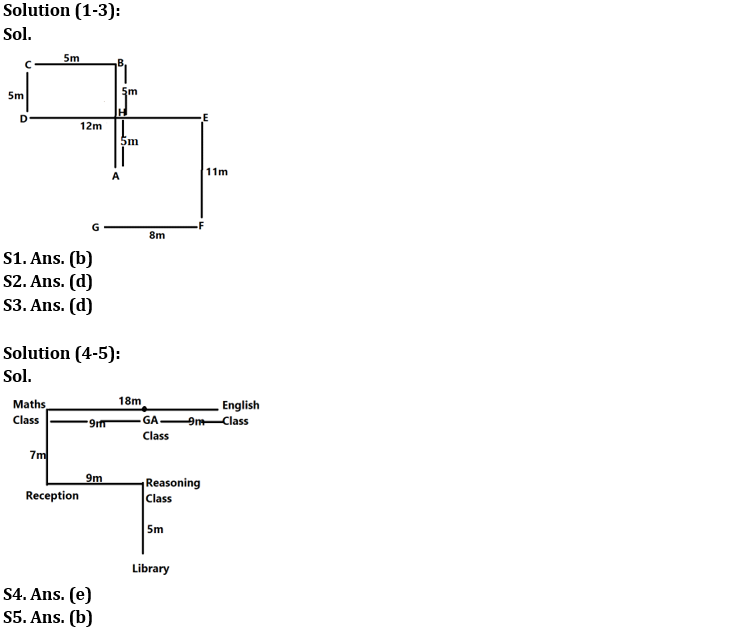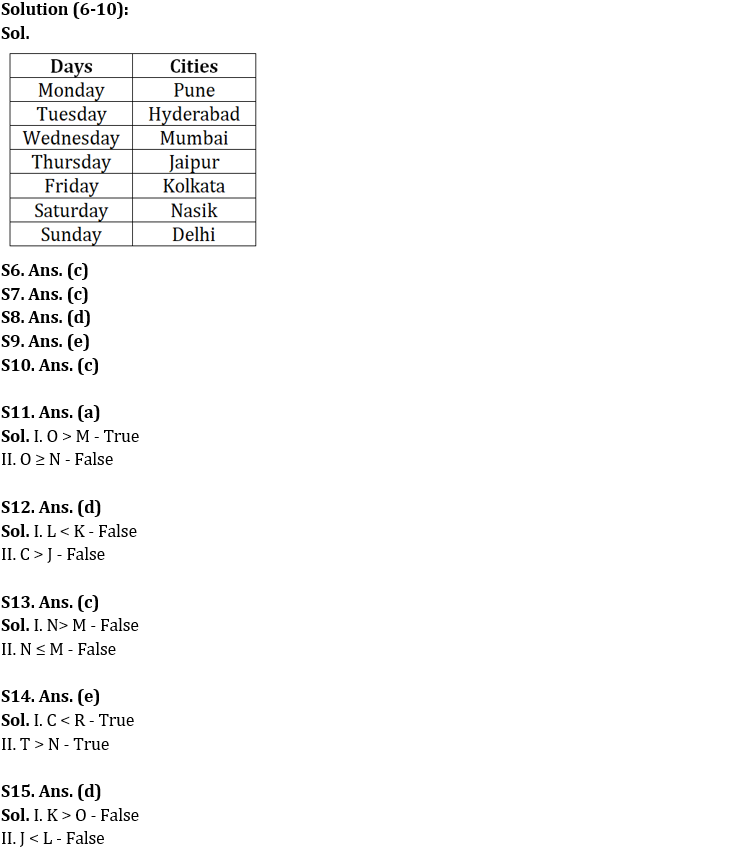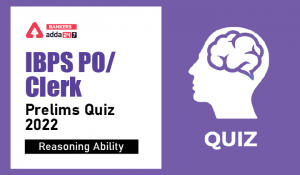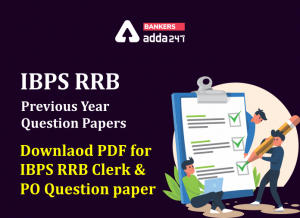Latest Banking jobs   »

# Reasoning Ability Quiz For IBPS RRB PO Clerk Prelims 2022- 25th July

Directions (1-3): Answer the questions based on the information given below:
Point B is 10m north of point A and 5m east of point C. Point E is 12m east of point D and 11m north of point F. Point C is 5m north of point D. Point G is 8m west of point F. Point H is 5m south of point B.

Q1. What is the direction of point G with respect to point B?
(a) North-west
(b) South-west
(c) North-east
(d) South-east
(e) None of the above

Q2. What is the shortest distance between point C and point E?
(a) 10m
(b) 11m
(c) 12m
(d) 13m
(e) None of the above

Q3. What is the direction of point F with respect to point H?
(a) North-west
(b) South-west
(c) North-east
(d) South-east
(e) None of the above

Directions (4-5): Answer the questions based on the information given below:
In an institute, Maths class is 18m west of English class. Reception is 7m south of Maths class. Reasoning class is 9m east of Reception and 5m north of Library. GA class is exactly in middle of Maths class and English class.

Q4. What is the shortest distance between Library and GA class?
(a) 18m
(b) 15m
(c) 17m
(d) 13m
(e) 12m

Q5. In which direction is Reception with respect to English class?
(a) North-west
(b) South-west
(c) North-east
(d) South-east
(e) None of the above

Directions (6-10): Read the following information carefully and answer the questions that follow:
Seven persons go to different cities among Mumbai, Delhi, Hyderabad, Kolkata, Jaipur, Pune and Nasik but not necessarily in the same order. Each of them goes via a flight which is scheduled for seven days from Monday to Sunday.
The person who goes to Pune is scheduled for Monday. Two persons are going between the person who goes to Kolkata and Hyderabad. The person who goes to Jaipur is scheduled after the person who goes to Mumbai. Only one person goes between the person who goes to Kolkata and Delhi. The person who goes to Jaipur is scheduled for Thursday. There are two persons scheduled between the person who goes to Mumbai and Nasik. The person who goes to Delhi is scheduled for three days after the person who goes to Jaipur.

Q6. Which combination is true regarding the given arrangement?
(b) Sunday – Pune
(c) Wednesday – Mumbai
(d) Tuesday – Kolkata
(e) Monday – Nasik

Q7. How many persons go between the person who goes to Mumbai and Delhi?
(a) One
(b) Two
(c) Three
(d) Four
(e) More than four

Q8. In which place a person goes immediately before the person who goes to Nasik?
(a) Delhi
(b) Jaipur
(c) Pune
(d) Kolkata

Q9. How many persons are scheduled after the person who goes to Hyderabad?
(a) One
(b) Two
(c) Three
(d) Four
(e) More than four

Q10. In which day a person goes to Nasik?
(a) Sunday
(b) None of these
(c) Saturday
(d) Tuesday
(e) Friday

Directions (11-13): In the following questions assuming the given statement to be true, find which of the conclusion(s) among given conclusions is/are definitely true and then give your answers accordingly.

Q11. Statements: O = G > M ≥ N < E, O > B, A = E
Conclusions: I. O > M
II. O ≥ N
(a) Only I is true
(b) Only II is true
(c) Either I or II is true
(d) Neither I nor II is true
(e) Both I and II are true

Q12. Statements: F = G > C ≥ K < E, F > J, L = E
Conclusions: I. L < K
II. C > J
(a) Only I is true
(b) Only II is true
(c) Either I or II is true
(d) Neither I nor II is true
(e) Both I and II are true

Q13. Statements: F = G > N ≥ D < M, F > B, O = M
Conclusions: I. N> M
II. N ≤ M
(a) Only I is true
(b) Only II is true
(c) Either I or II is true
(d) Neither I nor II is true
(e) Both I and II are true

Directions (14-15): In the following questions assuming the given statement to be true, find which of the conclusion(s) among given conclusions is/are definitely true and then give your answers accordingly.

Q14. Statements: T > R > Q > N; N > C; D < R
Conclusions: I. C < R
II. T > N
(a) Only I is true
(b) Only II is true
(c) Either I or II is true
(d) Neither I nor II is true
(e) Both I and II are true

Q15. Statements: G > K > L < H; G > O; H > J
Conclusions: I. K > O
II. J < L
(a) Only I is true
(b) Only II is true
(c) Either I or II is true
(d) Neither I nor II is true
(e) Both I and II are true

Solutions#### Congratulations!Incorrect details? Fill the form again here

•Reasoning Ability Quiz For IBPS RRB PO C...
•Quantitative Aptitude Quiz For IBPS RRB ...
•Reasoning Ability Quiz For IBPS Clerk/PO...
•Reasoning Ability Quiz For IBPS RRB PO C...
•Quantitative Aptitude Quiz For IBPS RRB ...
•IBPS RRB Previous Year Question Paper PD...